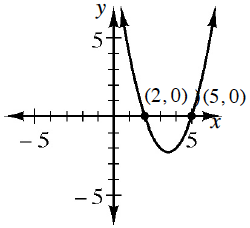### Home > CCAA8 > Chapter 11 Unit 12 > Lesson CCA: 11.3.4 > Problem11-121

11-121.

$\$This problem is a checkpoint for the quadratic web. It will be referred to as Checkpoint 11. Find the required missing part of the quadratic web.

1. Make an $x→y$ table and then draw a graph of                                                               $y=x^2−2x−3$.

1. Determine a possible equation for the given graph.1. Without making an $x→y$ table, find the $x$-intercepts of this quadratic. Use them to find the vertex and then sketch a graph.

$y=x^2+8x+7$

1. The height in feet of a rocket after $x$ seconds is given by $y=128x−16x^2$. What is the maximum height reached by the rocket and how long does it take to reach that height?

Check your answers by referring to the Checkpoint 11 materials located at the back of your book.

Ideally, at this point you are comfortable working with these types of problems and can solve them correctly. If you feel that you need more confidence when solving these types of problems, then review the Checkpoint 11 materials and try the practice problems provided. From this point on, you will be expected to do problems like these correctly and with confidence.

Answers and extra practice are located in the back of your printed textbook or in the Reference Tab of your eBook.
If you have an eBook for CCA, login and then click the following link: